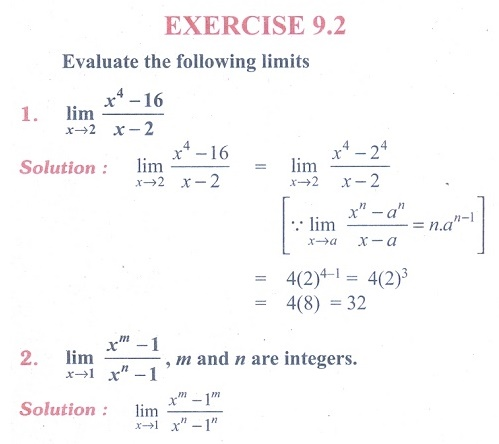Home | | Maths 11th std | Exercise 9.2: Theorems on limits

# Exercise 9.2: Theorems on limits

Maths Book back answers and solution for Exercise questions - Evaluate the following limits - Mathematics : Differential Calculus - Limits and Continuity: Theorems on limitsTags : Problem Questions with Answer, Solution | Mathematics , 11th Mathematics : UNIT 9 : Differential Calculus Limits and Continuity
Study Material, Lecturing Notes, Assignment, Reference, Wiki description explanation, brief detail
11th Mathematics : UNIT 9 : Differential Calculus Limits and Continuity : Exercise 9.2: Theorems on limits | Problem Questions with Answer, Solution | Mathematics